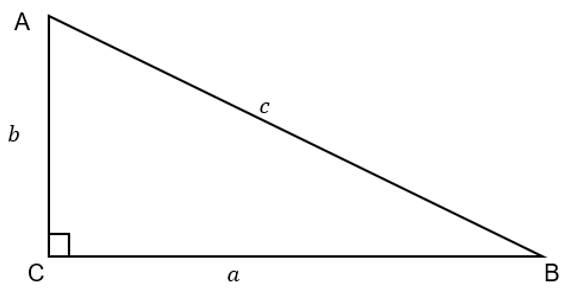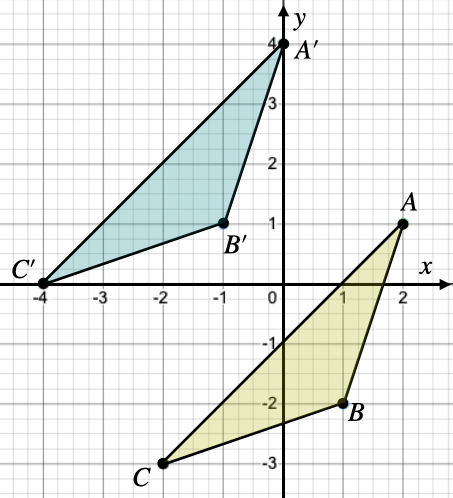8th grade math curriculums are fairly standard across the U.S., with minor variations from state to state. Inside this article you will find the major topics your children will be studying in 8th grade math. With this knowledge of the 8th grade math curriculum, you will be able to better understand what type of problems and topics your children will be working on in the 8th grade.

## 8th Grade Math Curriculum Topics (With Definitions)

### Irrational Numbers

Irrational numbers are numbers that cannot be expressed as a ratio and have an infinite non-recurring expansion when expressed as a decimal. Π (Pi) is an example of an irrational number. It goes on forever when expressed as a decimal i.e. 3.141592653… without ever repeating a pattern. It cannot be expressed as any simple ratio. A rational number would be something like 4/3. While when expressed as a decimal it does go on forever (1.3333333333…), it repeats the same pattern of 3s, unlike Pi which has no pattern. In 8th grade math students learn to work with irrational numbers.

### Laws of Exponents

An exponent is a quantity representing the power to which a given number or expression is to be raised, usually expressed as a raised symbol beside the number or expression (e.g. 3 in 2^3 = 2 × 2 × 2). In 8th grade math students learn to add, subtract, multiply and divide with exponents.

### Scientific Notation

Scientific notation is a way to express vast and tiny numbers as a product of 10 so that the number is easier to read. Proxima Centauri, the closest star to earth, is 40,208,000,000,000 km away. The mass of a hydrogen atom is 0.000000000000000000000000167 grams. These are some examples that are hard to read and work with. Scientific notation is a way to make these numbers easier to read. So the distance to Proxima Centauri would be written as 4.0208 x 10^13 and the mass of a hydrogen atom would be written as 1.67 x 10^-25

### Square Roots and Cube Roots

A square root is a number which produces a specified quantity when multiplied by itself.

7 is a square root of 49 because 7 x 7 is 49. The cube root of a number is a special value that, when used in a multiplication of itself three times, gives that number. For example, the cube root of 27 is 3 because 3 × 3 × 3 = 27. In 8th grade math students learn to work with square roots and cubed roots.

### Introduction to Systems of Equations

A System of Linear Equations is when we have two or more linear equations working together. A Linear Equation is an equation for a line on a graph. There are many instances in the real-world context where systems of linear equations can be applied. For example if you left the house at 2:00pm driving 30mph, but someone else left five minutes after you and was going 40mph, you could figure out how long it would take them to catch up with you using systems of linear equations.

### Functions

A function is a relation in which each element in the domain corresponds to one and only one element in the range. A relation is a rule that relates values of the first set of values (called the domain) to the second set of values (called the range).

### Evaluating Functions

Evaluating a function f(x) means finding the value of the function that corresponds to a given input value of x.

Example:

f(x) = 2x2 - 5x + 1

If x=3 then you would simply input the 3 in place of the x’s to solve the equation.

2(3)^2 - 5(3) + 1 = 4

This is an example of working with functions. In 8th grade math students learn to work with and solve more complex functions as well as graph functions.

### The Pythagorean Theorem

The Pythagorean Theorem is one of the most useful theorems in Geometry and Trigonometry. It has many applications in science and measurement involving right triangles. Its equation is a^2 + b^2 = c^2. It is used to find the area of right triangles and the length of its sides. In 8th grade math students learn to work with this theorem and use it to solve problems related to triangles.### Transformation of Images

There are four different types of transformations which are Dilations, Reflections, Rotations and Translations. A dilation is a type of transformation that resizes (stretches or shrinks) the original figure. A reflection is a type of transformation that flips a figure across a line. In rotation, a figure or image is turned around a center in a specific direction through a given angle. A translation moves (slides) or displaces every point of the figure by the same distance and in the same direction. This image is an example of a translation:In 8th grade math, students learn to work with these types of transformations of images on graphs and by using equations which show what type of transformation is occurring.

### Compound Probability

Probability is a measure of the likelihood of the occurrence of an event. The probability related to the occurrence of two or more events is called Compound probability. This is where students would learn how to calculate for example the likelihood of drawing a two and a seven from a deck of cards. In 8th grade math students work with more complex problems of probability than in previous years.

## How MathPrep Can Help

MathPrep is the ultimate math practice site. Our goal is to help children become confident math students and eliminate all math test anxiety forever. We do this by giving students what they need most to excel at math–practice.

MathPrep has thousands of practice problems and gives students the ability to take timed practice tests so they can be 100% prepared for their math tests. Our software tracks students progress and guides students to better abilities on a gradient of difficulty using cutting edge artificial intelligence.